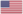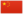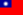# 虎星 (预言开始)

TigerstarTigerstar虎星虎星

Q712：黑森林公猫，原雷族/影族猫，自《预言开始》系列登场的角色

## 细节

### 外貌

• 他是一只身形高:108大、:1结实:150沉重、:8皮毛厚实:158的暗棕色虎斑公猫。他有着特别长:猫物表的尖锐:46前爪，:猫物表宽阔的胸脯:36和肩膀，:6以及淡:151琥珀色:3的大眼睛。:199他的右耳:72有一道从顶部开始的深V伤口，鼻梁上横过一道疤痕，:45皮毛上长长的战疤交错纵横。:256
• 他的外貌和祖先金雀掌十分相似。:496-497

### 趣闻

• 他曾被定名为铁锤掌Hammerclaw），但后来因为猫族应该不知道铁锤是什么，所以才被改成了虎掌。
• 在同窝手足中，他排行老三。:19章
• 他被命名为虎掌，是因为他那巨大，“令猫生畏”的脚爪。:猫物表
• 他的儿子黑莓星:6章和鹰霜的外貌和他即为相似。:10章
• 他的外孙虎星的名字是为了纪念他。:85

## 语录

—— 鹅羽的自言自语，关于虎爪，《蓝星的预言》，第265页

—— 虎掌企图谋杀蓝星，《疑云重重》正体中文版，第239页

—— 虎掌的想法，关于火心，《虎掌的愤怒》正体中文版，第1章

—— 虎掌的想法，在他负伤被放逐时，《虎掌的愤怒》正体中文版，第1章

—— 虎掌的想法，关于红尾，《虎掌的愤怒》正体中文版，第1章

—— 虎掌的想法，关于仁慈，《虎掌的愤怒》正体中文版，第4章

—— 虎掌和影族猫，关于他离开雷族，《虎掌的愤怒》正体中文版，第54页

—— 虎星对火星，在血族第一次出现的会谈上，《力挽狂澜》，第22章

—— 虎星对黑莓掌，《日落和平》，第250页

—— 虎星对狮爪，《暗河汹涌》，第331页

—— 斑叶警告松鸦羽不得轻视虎星，《战声渐近》，第217页

—— 虎星和藤爪，关于虎心和焰尾，《暗夜密语》正体中文版，第279页

—— 藤爪的想法，当虎星对焰尾的死感到庆幸时，《暗夜密语》正体中文版，第279页

## 登场书目

• 呼唤野性 (Q57)[主要角色][视角人物][首次登场🐱]
• 寒冰烈火 (Q59)[配角]
• 疑云重重 (Q60)[配角]
• 风起云涌 (Q65)[配角]
• 险路惊魂 (Q66)[配角]
• 力挽狂澜 (Q67)[主要角色][视角人物][死亡时刻✝]
• 午夜追踪 (Q139)[对话提及]
• 新月危机 (Q140)[对话提及]
• 重现家园 (Q141)[对话提及]
• 星光指路 (Q142)[配角]
• 黄昏战争 (Q143)[配角]
• 日落和平 (Q144)[配角]
• 预视力量 (Q152)[配角]
• 暗河汹涌 (Q153)[配角]
• 驱逐之战 (Q154)[配角]
• 天蚀遮月 (Q155)[配角]
• 暗夜长影 (Q156)[配角]
• 拂晓之光 (Q157)[配角]
• 第四学徒 (Q158)[配角]
• 战声渐近 (Q159)[配角]
• 暗夜密语 (Q160)[配角]
• 月光印记 (Q161)[对话提及]
• 武士归来 (Q162)[配角]
• 群星之战 (Q163)[主要角色][死亡时刻✝]
• 学徒探索 (Q170)[对话提及]
• 雷影交加 (Q171)[间接提及]
• 烈焰焚河 (Q174)[对话提及]
• 风暴来袭 (Q175)[对话提及]
• 迷失群星 (Q3563)[对话提及]
• 叠影重障 (Q3624)[对话提及]
• 无星之地 (Q4385)[对话提及]
• 火星的探索 (Q176)[对话提及]
• 蓝星的预言 (Q177)[配角]
• 黄牙的秘密 (Q180)[对话提及]
• 黑莓星的风暴 (Q182)[对话提及]
• 蛾飞的幻象 (Q183)[仅于幻象]
• 鸦羽的拷问 (Q186)[对话提及]
• 灰条的誓言 (Q4057)[对话提及]
• 豹星的荣耀 (Q4426)[配角][死亡时刻✝]
• 雾星的征兆 (Q188)[对话提及]
• 虎掌的愤怒 (Q190)[主人公][主要角色][视角人物]
• 叶池的祈愿 (Q191)[对话提及]
• 鸽翅的沉默 (Q192)[对话提及]
• 乌爪的告别 (Q195)[对话提及]
• 斑叶的衷肠 (Q196)[配角]
• 松星的抉择 (Q197)[配角]
• 红尾的恩债 (Q3565)[主要角色]
• 褐皮的族群 (Q3566)[对话提及]
• 蛾翅的秘密 (Q3628)[对话提及]
• 黑脚的清算 (Q4336)[仅于回忆]
• 斑毛的叛变 (Q4337)[对话提及]
• 进入森林 (Q210)[主要角色]
• 逃出森林 (Q211)[主要角色]
• 返回族群 (Q212)[主要角色]
• 和平破碎 (Q213)[对话提及]
• 武士之心 (Q215)[对话提及]
• 长鞭崛起 (Q206)[配角][死亡时刻✝]
• 河族阴云 (Q4311)[仅于回忆]
• 变革之风 (Q4411)[对话提及]
• 族群的秘密 (Q199)[对话提及]
• 族群的猫 (Q200)[配角]
• 族群的守则 (Q201)[配角]
• 族群的战争 (Q202)[配角]
• 终极指南 (Q204)[视角人物]
• 长老的忧虑 (Q4324)[对话提及]
• ## 外部链接

### 官方文章

• 火之传奇 （提及）
• 族群中的领导者们 （提及）
• 名垂青史的族长们 （隐射）
• 《迷失群星》诞生记 （提及）
• 向猫妈妈致敬 （提及）
• 选个绒毛娃娃：第二回 （投票选项）
• 第一场九命仪式 （提及）
• 不容忽视的“爪字辈”们 （提及）
• 脱颖而出 （提及）
• 我们捕猎为生 （提及）
• 新猫页面－褐皮 （提及）
• 武士守则：过时的遗俗？ （提及）
• 生活不止一种 （提及）
• 邪恶永存 （提及）
• 至尊反派 （专题）
• 创造反派 （提及）
• 团结则存，分裂则亡 （提及）
• 共同的纽带 （提及）
• 预料之外的剧情 （提及）
• 你至亲的至亲 （提及）
• 当征兆为假 （提及）
• 我们的边界之外 （提及）
• 解读征兆 （隐射）
• 禁忌之爱 （提及）
• Nothing But Stories （隐射）
• Asking a Great Deal （提及）

## 脚注

1. 原因：未指派 (Q4296) - 未指派特定人物负责该职务，临时协助顶替
2. 原因：黑森林训练 (Q4420) - 活猫进入无星之地并接受训练

## 参考文献

这篇文章基于CC BY-SA 3.0许可使用了猫武士维基（英语）Tigerstar (TPB)一文中的部分内容。
1. 在同一份由维琪授意、Su所公布的黑森林名单中，雪暴翅、亮花和沼云已在官网家谱被标注为星族成员；鹿脚、百合须、短尾和缠果的死后去处则暂时没有更进一步的资讯。
2. 在和星族失联后，死去的族群猫灵魂都被蜡毛控制并囚禁于黑森林中。
5.0
1人评价